ISSN: 0970-938X (Print) | 0976-1683 (Electronic)

# Biomedical Research

An International Journal of Medical Sciences

- Biomedical Research (2016) Volume 27, Issue 2

## Quality assessment of NIR finger vascular images for exposure parameter optimization.

 Michał Waluś1, Krzysztof Bernacki2*, Adam Popowicz3 1Faculty of Automatic Control, Electronics and Computer Science, Silesian University of Technology, Akademicka 16, 44-100 Gliwice, Poland 2Institute of Electronics, Faculty of Automatic Control and Computer Science of the Silesian University of Technology, Akademicka 16, 44-100 Gliwice, Poland 3Institute of Automatic Control, Faculty of Automatic Control and Computer Science of the Silesian University of Technology, Akademicka 16, 44-100 Gliwice, Poland Corresponding Author: Krzysztof Bernacki, Institute of Electronics Silesian University of Technology Poland Walus/Bernacki/Popowicz 390/td> Accepted: February 28, 2016
Visit for more related articles at Biomedical Research

### Abstract

The measurement of image quality plays an important role in all acquisition systems. In many medical applications, such as in the presented Near Infrared (NIR) imaging of the finger vascular system, there is no possibility of comparing the system outcomes with a reference data set. As a solution for this problem, a range of available methods have been presented, which try to reflect or imitate subjective human operator assessment. In this paper, we present a review of such quality metrics and introduce a novel approach based on distance transformations. The proposed method was compared with the state-of-the-art metrics on NIR vascular system images obtained by our constructed acquisition device. By changing the intensity of the finger backlight and by examining intentionally blurred images, we proved that our approach was capable of providing most sensible quality outcomes, while the others turned out to be much less reliable. The proposed evaluation technique may also be employed in any other medical application, where the assessment of the image quality has a direct impact on the final diagnosis.

### Keywords

Biomedical image processing, Finger vascular system, Image quality, Image enhancement.

### Introduction

Finger vascular recognition has been met with growing interest in the last few years. The available devices for biometric sample acquisition (research prototypes as well as commercial solutions) are based on lighting the finger by light-emitting near-infrared diodes (NIR-LED) and capturing images depicting the vein and artery structures. These structures are subcutaneous, thus they cannot be observed by the naked eye.
Recently, several research centers worldwide have proposed diverse investigations to use finger vascular patterns for biometric recognition [1-9]. The first suggestion to use the blood vessel network as a biometric characteristic was made more than a decade ago  since then, a large number of different techniques for finger vein image acquisition and its preprocessing for the purpose of quality enhancement have been developed. In contrast to the other human identification methods, which involve face characteristics or fingerprints, the NIR vascular system recognition is more robust against spoofing because it requires the subject to both be alive and give permission.
Finger vascular imaging is also used in an increasing number of new medical applications and is an alternative method to widespread imagery techniques, such as thermography, Doppler laser , plethysmography  or capillaroscopy . NIR imaging methods do not require ionizing radiation and have great potential for, e.g., diagnosis of articular cartilage pathology  or breast cancer diagnosis . In contrast to X-ray techniques, it is not harmful to living tissues, thus it may be employed with no danger to the patients.
The main requirement for the correctness of recognition or the accuracy of the medical diagnosis is the highest possible quality of the images. To make the imaging system efficient and reliable, several hardware parameters, such as the backlight intensity or the exposure time, have to be optimized in the initial stage of the acquisition process. The image quality metrics are the main coefficients that control these optimization procedures.
In this paper, we compare several image quality evaluation methods, and we also present a novel approach based on distance transformations. Our algorithm allows for the selection of optimal exposure parameters, thus the amount of information included in a given image is maximized. We also present the usability of NIR imaging for a biometric acquisition system. For such an application, the image enhancement leads to improvement in the identification. However, it should not be forgotten that the presented technique is also usable for all NIR-based imaging techniques, where there is no possibility of obtain the reference frame for the quality assessment.
The paper is organized as follows. In Section 2, we present and describe the biometric authentication system with the image assessment employed. Next, in Sections 3 through 5, we enumerate and provide the basics of the state-of-the-art image quality metrics. In Section 6, our novel approach is introduced. Finally, we present the details of our experiments in Section 7, and we discuss the results in Section 8.

#### Biometric authentication with image quality evaluation

In the human body, veins and arteries have different thicknesses and sizes. It is well-known that various tissues of a finger are characterized by different absorption coefficients . This phenomenon can lead to the loss of information regarding the vascular structure due to over- or under-exposure in some parts of an acquired NIR finger image. In most practical applications, the range of possible image grayscale values are stored in an 8-bit dynamic range (0~255). Therefore, the values outside this range cause a loss of quality, which can be recovered by reducing the intensity level of the LED-matrix brightness or decreasing the exposure time. Therefore, the acquisition system should assess the camera “snapshots” and register only those images that are not saturated.
A block diagram of the biometric authentication system is presented in Figure 1. The acquisition process focuses on the hardware issues, wherein the system optimizes the exposure parameters to reach the highest image quality. Adjustment of the acquisition system parameters (Hardware Tuning) can be performed by modifying the backlight intensity, zooming, autofocusing, filtering by employing different optical pass filters, stabilizing the camera, etc. The mentioned techniques aim for the improvement of the image quality before storing the frames. Depending on the system design, the parameters may be tuned automatically or manually. The process is performed iteratively in a feedback loop, wherein the quality of the obtained images is estimated in real-time therefore, the selection of a proper quality metric is crucial for the overall efficiency of the biometric authentication system.
The second part of the presented system, the software image pre-processing, includes the algorithms that highlight all the desirable image content to be further investigated during the feature extraction. In this stage, the image adjustment is based on reshaping the histograms, scaling, sharpening, removing the noise, applying morphological operations, etc.

#### Double-indicator image quality evaluation method

The quality estimation system, presented in , combines information provided by two statistical descriptors, mean and variance, which allows for the calculation of the evaluation score, called the double-indicator. The first descriptor, the mean intensity, reflects the overall image brightness, while the second one, the variance of the intensity, corresponds to the distribution of pixel values and may also be utilized as an indirect measure of image contrast. The descriptors are given by the following formulas: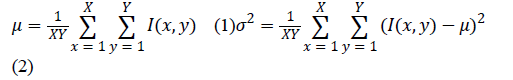where I(x, y) is the intensity of the pixel at position (x, y) and X and Y stand, respectively for the image width and height.
The quality evaluation score of an image, the double-indicator (D), is defined as follows: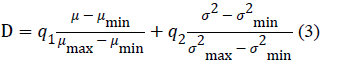where μmin and μmax are the minimum and maximum gray mean of each image line and σ2 and σ2max are the minimum and maximum gray variance of each image line. In this paper, it is assumed that the weights q1 and q2 have the same value, 0.5.
The variance itself also provides information about the image contrast. Therefore, in some applications, the double-indicator is reduced only to the square root of its second component. In such a case, the standard deviation (σ) of the pixel intensities is maximized to obtain the optimal image quality.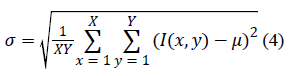#### Maximization of image entropy

Another method of quality evaluation calculates entropy to investigate the average amount of information included in each image pixel . It considers the image as an experiment, where the pixels intensities are the states with given probabilities. Assuming that pixels values are variables that may receive values from k=1,2,…,K, the image entropy is given by: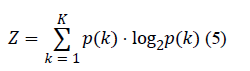where p(k) is the probability of the appearance of intensity k.
The presented method was utilized for the assessment of NIR images in . By modifying the intensity of the LED matrix emission, the authors optimized the acquisition parameters to reach the highest entropy of the obtained NIR vascular images. The experiments proved the usability of the employed evaluation approach, and their closed-loop system showed good stability and fine convergence.

#### Co-occurrence matrix and image descriptors

The co-occurrence-based method takes into account the information of pixels’ spatial distribution. The co-occurrence matrix is a square K × K matrix, where K is the maximal value of pixel intensity (e.g., for an 8-bit image, K=256). Each (m, n) element of the co-occurrence matrix defines how many times a pixel with intensity level m appears with a neighboring pixel of intensity n.
Figure 2 depicts the basics of filling the co-occurrence matrix. For example, the element of the co-occurrence matrix at (1,1) is set as 1 because there exists only one occurrence of neighboring 1-1 values in the image matrix. Similarly, the element at (1,2) is 2 due to the presence of two 1-2 pairs in the image. An exemplary co-occurrence matrix calculated for a complex grayscale image is given in figure 3. It should be noted that the values on the diagonal of the co-occurrence matrix correspond to smooth parts of the image, while the further from the diagonal, the higher the intensity gradients become.
After normalization, the elements of the co-occurrence matrix wmn can be interpreted as the probabilities of transitions between the intensity levels in the image. For an image of X × Y size, the normalized elements of the co-occurrence matrix are calculated as follows: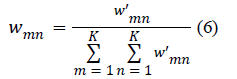where w’mn is the element at (m, n) in the co-occurrence matrix, and wmn is the corresponding element after normalization.
The co-occurrence matrix contains a large amount of information regarding the image characteristics. The quality measurement of vascular patterns, which uses global and local features computed based on a Gray Level Co-Occurrence Matrix, can be found in . In our comparison study, we utilized the following coefficients specified in : the uniformity of energy (also called the energy) (7), the contrast (8), the correlation (9) and the homogeneity (10).
Image characteristics designated based on the normalized cooccurrence matrix:
Uniformity of energy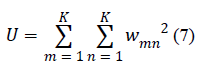Contrast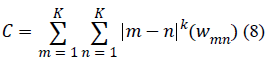Correlation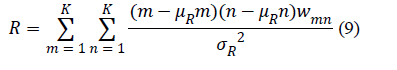where: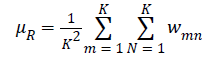and Homogeneity (10)Distance transformation-based image quality
Distance transformations were originally introduced by Rosenfeld and Pfaltz , for the quick estimation of metric distance between the indicated image object and the other pixels. The distances are calculated with a use of the so-called double-scan algorithm and stored in a distance matrix. Initially, all the pixels except for the object pixels are assigned an infinite distance (Figure 5b). Then, a local mask slides over the distance map twice: from left to right, top to bottom, and then again from right to left, bottom to top. This double-scan process is visualized in figure 4. At each step during the sliding, very simple replacement operations are performed within the mask (Figure 4, gray):
dA=min {dpi+1, dA} (11)
where dA and dPi are the distance values at the mask pixels A and Pi, respectively, and min{} denotes the minimum value. An example of applying the distance transformations to estimate the distances from a simple 3-pixel object is presented in figure 5 note that, after the first scan, only a subset of pixels receive their distances (Figure 5c). Eventually, after the second scan, the entire distance matrix is populated with the distance estimations (Figure 5d).
To obtain the quality of a given image, we utilized the idea of distance transformations with a simple extension. Such an approach was also introduced for colorization purposes in [23-25]. Instead of analyzing the metric distances between neighboring pixels, we focus on the intensity differences. Hence, Equation-(11) in our method is modified in the following way:
dA=min{|IA-IPi|, dA} (12)
where IA and IPi are the intensities of the pixels A and Pi, respectively, and | · | denotes the absolute value. Therefore, the resulting distance matrix reflects the magnitude of intensity gradients encountered between pixels, so it also measures the number of structure fluctuations visible in the image. Hence, we assumed that this method may be successfully employed as the image quality measure.
The most valuable information retrieved from the NIR images of the finger vascular system is located in the darker parts associated with the presence of veins and arteries. Therefore, to make the algorithm better suited for our NIR exposures and to make it sensitive to visibly darker objects in the image, we decided to extend Equation-(12), so that we include only the intensity growth in the distance measure:
dA=min{α. |IA-IPi|, dA} (13)
where α is our distance trigger, defined as follows:
α=1 if IA-IPi <0, otherwise α=0 (14)
To obtain the quality of an image, we first calculate the socalled local quality in each image pixel. To do so, we analyze the pixel’s N × N neighborhood window employing our extended distance transformation. The pixels intensity distances (di) determined from the window’s central pixel allow for the calculation of the mean distance (μd), and the number of pixels with positive distance (Cd). These two coefficients represent, respectively, the qualitative and the quantitative estimations of pixel degree of the membership to the vascular system (i.e., the larger the Cd or μd, the more probable it is that the pixel depicts a part of the vein). The multiplication of the coefficients yields the local quality measure (Ql). The process of retrieval of local quality at an exemplary pixel is presented in figure 6. In figure 6f, we show the formulas and the results of the calculation of the mean distance (μd), the number of pixels with positive distance (Cd) and the final local quality measure (Ql).
That process is performed for each image pixel, such that we obtain a local quality map that highlights the most informative parts of the image. Then, the mean of the Ql values over the entire image matrix is selected as our overall quality measure (Q).
Examples of local quality maps for two NIR images are depicted in figures 7b and 7d. The overall quality results Q are also provided.
The size of the window utilized in the local quality estimation should be chosen properly with respect to a given object of interest. While small windows focus on fine details, larger ones are better suited to extended structures. As a rule of thumb, one should choose a window at least as large as the smallest size of observed objects (e.g., for veins, it should be their width). Several examples of local quality maps obtained with different mask sizes are depicted in Figure 8.

### Experiments

To perform all required experiments, we have constructed a dedicated laboratory device that utilizes the infrared light transmission through the finger. We employed a CCD camera, which stored the results in a secured database. The utilized light source consisted of a self-constructed LED matrix to provide uniform and adjustable illumination .
In the first experiment, a set of NIR finger images for 20 levels of backlight intensities were collected from two volunteers. In figure 9 and figure 11, every second image from the entire series (20 measurements) is depicted. In figure 10 and figure 12, we present the results of the quality measurement using the seven described estimators and provide the outcomes of our proposed method. The second experiment exposed the results of quality coefficients for frames with an added blurring effect obtained by intentional finger motion during the exposure (Figure 13 and Figure 15). Only one of the 6 included images was not affected by the blurring, and it was positioned as the first from the left. In figure 18, we present a summary of our experiments, wherein, for each metric, we present the image indicated as that with the highest quality.
The optimal exposures, as selected using different estimators, showed an unexpected variety. In the first experiment, most of the methods indicated strongly saturated images as the sharpest frames. On the opposite side, the homogeneity maximal value was achieved for nearly black frames, wherein no finger details could be spotted. Only the entropy seemed to make reasonable image selection decisions. However, the results of our method showed definitely the best compromise between saturation and underexposure.
It is also worthwhile to compare the sensitivity of the quality measures by the assessment of the dynamic range of the optimization curves. For the proposed technique such a curve starts from Q=0 and peaks at approximately Q=120 (Figure 10 and Figure 12), while, e.g., for the entropy, the curve is within the 0.48~0.54 range. The dynamic range is a significant factor in the hardware tuning loop (Figure 1) because it improves the convergence process of the exposure parameter optimization.
In the experiment with 6 images, within which only one was not blurred, all the methods except for the proposed one indicated the wrong frames (Figure 14 and Figure 16). Even the entropy, which provided sensible outcomes in the previous experiment, was not able to recognize the optimal, sharp exposure. Such results disqualify the well-known methods as indicators of image selection in a practical implementation of a finger NIR acquisition system. Again, only our method made the correct selection.
The entropy and standard deviation, which provided reasonable quality estimations in the experiment 1, need however some more comments. They both are not dependent upon the spatial distribution of pixels within the image.
As an example, in figure 15 and figure 17, we show the randomization of pixel positions (the pixels were reshuffled), which entirely converts the imaging scene, while the calculated metrics remain unchanged.
It is a significant drawback of these methods, as they may not be employed simultaneously for a wide variety of observed objects and are usually useless for the assessment of images affected by any type of noise.
Summarizing, the presented algorithm appeared to be the most reliable when compare with many, widely spread, image quality measures.
It proved its superiority in the NIR finger vein image processing pipeline, where used as the indicator of the optimal exposure time and the estimator of image sharpness.

### Conclusion

The quality of captured images is an important premise for a variety of applications. In this paper, we emphasized the importance of the quality estimation of NIR finger images in the hardware tuning process, wherein the image assessment plays a crucial role during the adjustment of exposure parameters. We presented a review of known methods and introduced a novel technique of image quality evaluation. The statistical-based (entropy, standard deviation, double indicator) and the co-occurrence-based (contrast, correlation, homogeneity, energy) methods were compared with the proposed distance transformation approach. The results of the experiments with blurred and with over- or underexposed frames indicated that all of the state-of-the-art methods are inefficient when employed on NIR finger vascular system images. Only the proposed method proved to be capable of providing reasonable results and was able to recognize the images that were not blurred by the intentional finger motion. Additionally, a wide dynamic range of the proposed quality coefficient is an important aspect, which should improve the convergence of the hardware tuning loop. The proposed method may be utilized in the assessment of any type of premedical diagnostic images, such as in thermography, plethysmography or capillaroscopy. Additionally, due to its ability to indicate the regions of interest, the method may be successfully applied in various image segmentation tasks. This will be the main aim of our studies in the immediate future.

### Acknowledgment

Michał Waluś is a DoktoRIS holder for the scholarship program for innovative Silesia. This project was co-financed by the European Union under the European Social Fund. Adam Popowicz was supported by the Polish National Science Center, grant no. 2013/11/N/ST6/03051: Novel Methods of Impulsive Noise Reduction in Astronomical Images. The calculations were carried out using IT infrastructure funded by the GeCONiI project (POIG.02.03.01-24-099/13). This work was supported by the Ministry of Science and Higher Education funding for statutory activities (BK/227/ RAU-1/2015/10).

### Tables at a glance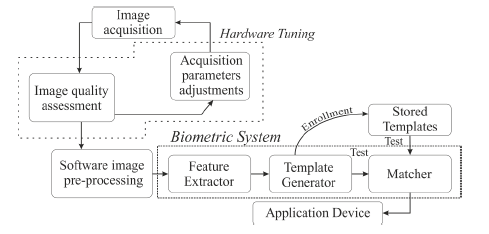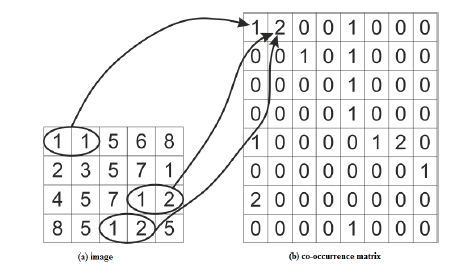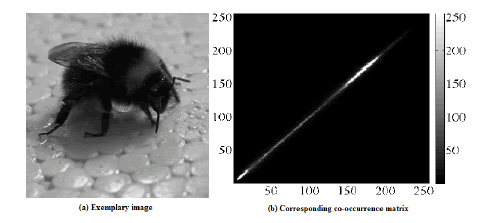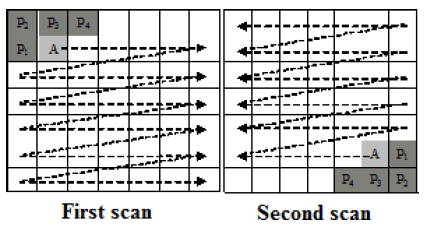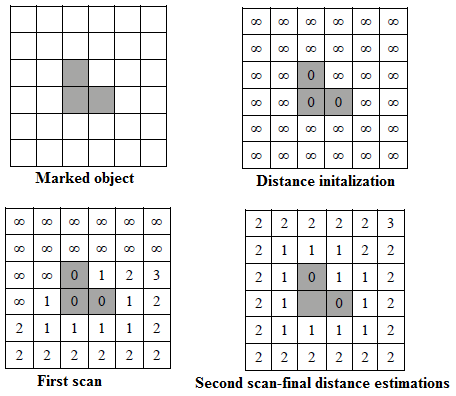Figure 1 Figure 2 Figure 3 Figure 4 Figure 5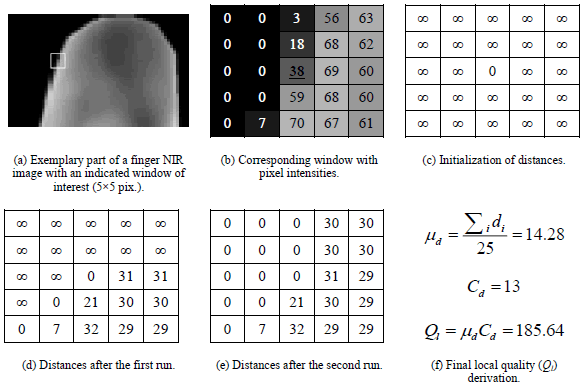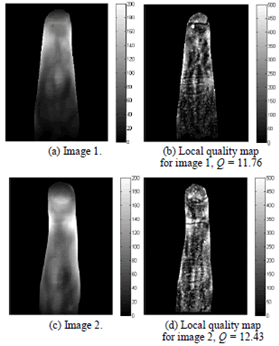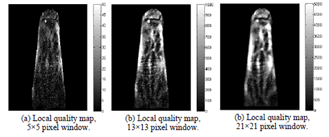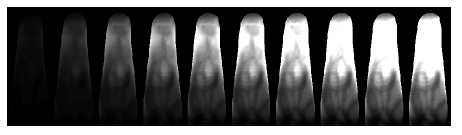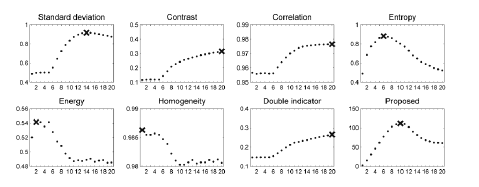Figure 6 Figure 7 Figure 8 Figure 9 Figure 10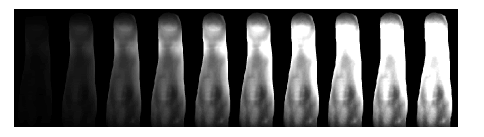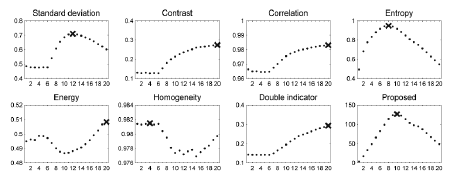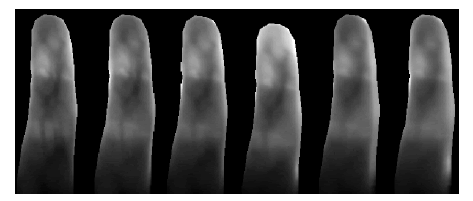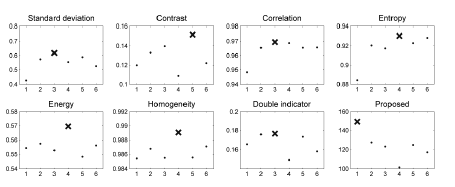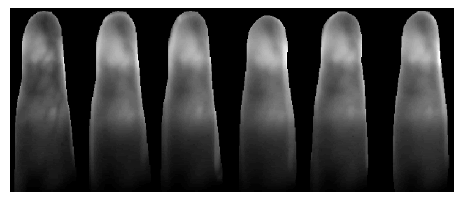Figure 11 Figure 12 Figure 13 Figure 14 Figure 15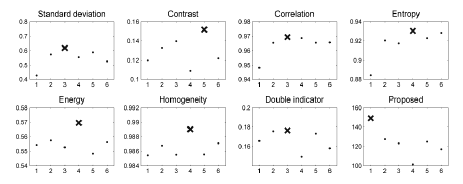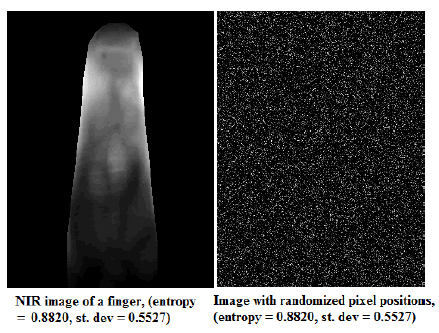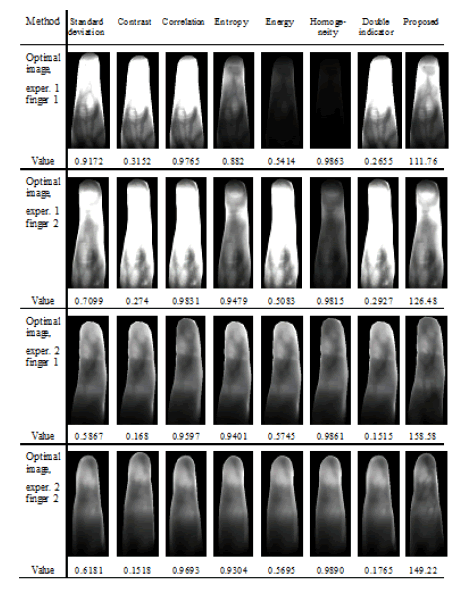Figure 16 Figure 17 Figure 18